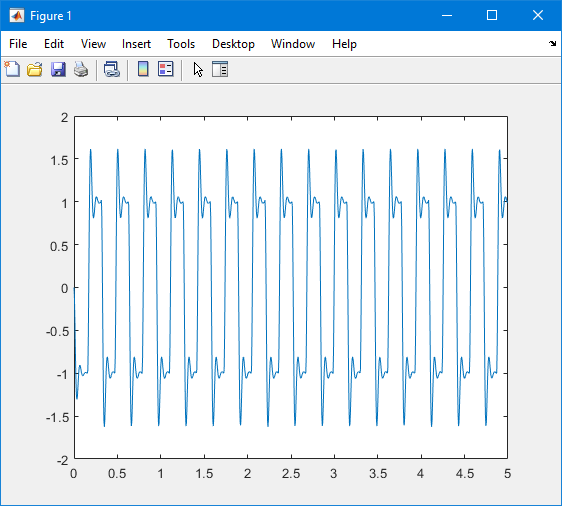## Plot Signal Data Logged to File by Simulink Desktop Real-Time

You can use the MATLAB® plotting functions for visualization of your non-real-time simulated data or your real-time executed data logged by Simulink® Desktop Real-Time™.

After running your real-time application and logging data to a file, you can plot the data.

This procedure uses the model `sldrtex_model`. To open this model, in the MATLAB Command Window, type:

```open_system(docpath(fullfile(docroot,'toolbox',... 'sldrt','examples','sldrtex_model')))```

The procedure assumes that you saved your data to the variable `ScopeData`.

The steps in this procedure assume that you have already loaded that model and have completed the steps in Set Scope Parameters for Logging to File, Set Run in Kernel Mode Properties for Logging to File, and Set Run in Kernel Mode Data Archiving Parameters.

1. In the Simulink Editor, on the Desktop Real-Time tab, click Run in Real Time.

When the real-time application runs, it creates the `ScopeData` variable that you configured in Set Run in Kernel Mode Properties for Logging to File and creates the archive data files that you configured in Set Run in Kernel Mode Data Archiving Parameters.

2. In the MATLAB Command Window, type:

```ScopeData ```
```ScopeData = time: [10000x1 double] signals: [1x1 struct] blockName: 'sldrtex_model/Scope' ```
3. To list the MAT-files saved to your disk, type:

```dir *.mat ```
```data_0.mat ```
4. To clear the MATLAB workspace and load the scope data, type:

```clear load data_0 who ```
```Your variables are: ScopeData ```
5. To plot the first 1000 points, type:

```plot(ScopeData_0.time(1:1000), ... ScopeData_0.signals.values(1:1000))```

The MATLAB environment plots the first `1000` samples over `0.0000–0.9990` seconds.